Life In 19x19http://lifein19x19.com/ KataGo @ Igo Hatsuyoron 120http://lifein19x19.com/viewtopic.php?f=18&t=16995 Page 4 of 6

 Author: Bill Spight [ Mon Jul 20, 2020 12:10 pm ] Post subject: Re: KataGo @ Igo Hatsuyoron 120 Cassandra wrote:Knowing the current status of Black's Main Semeai Liberties at every moment of the game is mandatory to identify "natural looking" moves as mistakes.I thought that this knowledge would be helpful, at least at amateur (Kyu) level.Excellent point! And not just at the kyu level. I think that a major reason for the relative weakness of today's bots for semeai is their inability to count as humans do.

 Author: Cassandra [ Mon Jul 20, 2020 11:47 pm ] Post subject: Re: KataGo @ Igo Hatsuyoron 120 Now, let' go back some moves, until Black's atari in the lower left. Click Here To Show Diagram Code`[go]\$\$Bcm115 SEVEN\$\$ +---------------------------------------+\$\$ | . . . X O . . . . . . . X X . . O X . |\$\$ | . . . X X O O X O O . X O X O . . O . |\$\$ | . . . X O X O O . . . X O O . O O . . |\$\$ | . X O , . X X . . O . X . O O X . . . |\$\$ | . . X X X X O O O . O X X . X X O O . |\$\$ | . X . O O O X X X O . . X . X O . O . |\$\$ | O O O O O X O O X X O O X O X . . X . |\$\$ | O X . X . X . O O X O . . . X X X X X |\$\$ | O X . X X . X O X X O . X X X X . O O |\$\$ | X O O X . X O O X O O X X O O O O 0 . |\$\$ | X X O O X O . O X O . X O O O . X X X |\$\$ | X X . O X . . O X O . X O O X X X O O |\$\$ | X X O O X . . O X O . X O . O . X X O |\$\$ | X O O a O X . O X O . X X O O O X O O |\$\$ | X O X O 9 X . O X O X . O X X X O O O |\$\$ | O X X X X X . O X O O O O X O X O O X |\$\$ | O . . . O X O O X X X X X . O X X O X |\$\$ | O . X . X O . O O O O O O X O X . O X |\$\$ | . X . . X O O O . . . . O X X X . O . |\$\$ +---------------------------------------+[/go]`White remembers that it is not mandatory to connect her two stones with a move at a, but plays her move 124 in the hanezeki, stopping Black's kikashi there.White will win the Semeai Variation by at least three points (@ komi=1). Click Here To Show Diagram Code`[go]\$\$Bcm123 SEVEN\$\$ +---------------------------------------+\$\$ | . . . X O . . . . . . . X X . . O X . |\$\$ | . . . X X O O X O O . X O X O . . O . |\$\$ | . . . X O X O O . . . X O O . O O . . |\$\$ | . X O , . X X . . O . X . O O X . . . |\$\$ | . . X X X X O O O . O X X . X X O O . |\$\$ | . X . O O O X X X O . . X . X O . O . |\$\$ | O O O O O X O O X X O O X O X . . X . |\$\$ | O X . X . X . O O X O . . . X X X X X |\$\$ | O X . X X . X O X X O . X X X X 2 O O |\$\$ | X O O X . X O O X O O X X O O O O 1 . |\$\$ | X X O O X O 7 O X O . X O O O . X X X |\$\$ | X X . O X 5 6 O X O . X O O X X X O O |\$\$ | X X O O X a . O X O . X O . O . X X O |\$\$ | X O O 4 O X . O X O . X X O O O X O O |\$\$ | X O X O 3 X . O X O X . O X X X O O O |\$\$ | O X X X X X . O X O O O O X O X O O X |\$\$ | O . . . O X O O X X X X X . O X X O X |\$\$ | O . X . X O . O O O O O O X O X . O X |\$\$ | . X . . X O O O . . . . O X X X . O . |\$\$ +---------------------------------------+[/go]`This implies that Black has to prevent White's move in the hanezeki by playing B 123 at this point himself in sente (White will not tenuki to cut at a in the centre), before inserting his second kikashi in the lower left.Again, the final scores will become W+2 / W+2 (@ komi=1). Click Here To Show Diagram Code`[go]\$\$Bcm123\$\$ +---------------------------------------+\$\$ | . . . X O . . . . . . . X X . . O X . |\$\$ | . . . X X O O X O O . X O X O . . O . |\$\$ | . . . X O X O O . . . X O O . O O . . |\$\$ | . X O , . X X T . O . X . O O X . . . |\$\$ | . . X X X X O O O . O X X . X X O O . |\$\$ | . X . O O O X X X O . . X . X O . O . |\$\$ | O O O O O X O O X X O O X O X . . X . |\$\$ | O X . X . X T O O X O . . . X X X X X |\$\$ | O X . X X . X O X X O . X X X X O O O |\$\$ | X O O X . X O O X O O X X O O O O X . |\$\$ | X X O O X . X O X O . X O O O . X X X |\$\$ | X X . O X X O O X O . X O O X X X O O |\$\$ | X X O O X . . O X O . X O . O . X X O |\$\$ | X O O O O X . O X O . X X O O O X O O |\$\$ | X O X O X X . O X O X . O X X X O O O |\$\$ | O X X X X X . O X O O O O X O X O O X |\$\$ | O . . . O X O O X X X X X . O X X O X |\$\$ | O . X . X O . O O O O O O X O X . O X |\$\$ | . X . . X O O O . . . . O X X X . O . |\$\$ +---------------------------------------+[/go]`What is eventually remaining in our "no kikashi" line?Black still has his kakashi at the top that compensates for White's newly found ko threat in the left centre. However, fighting Dany's ko was hopeless for Black even before.

 Author: Cassandra [ Thu Jul 23, 2020 11:03 pm ] Post subject: Re: KataGo @ Igo Hatsuyoron 120 Cassandra wrote:Now, let' go back some moves, until Black's atari in the lower left. Click Here To Show Diagram Code`[go]\$\$Bcm115 SEVEN\$\$ +---------------------------------------+\$\$ | . . . X O . . . . . . . X X . . O X . |\$\$ | . . . X X O O X O O . X O X O . . O . |\$\$ | . . . X O X O O . . . X O O . O O . . |\$\$ | . X O , . X X . . O . X . O O X . . . |\$\$ | . . X X X X O O O . O X X . X X O O . |\$\$ | . X . O O O X X X O . . X . X O . O . |\$\$ | O O O O O X O O X X O O X O X . . X . |\$\$ | O X . X . X . O O X O . . . X X X X X |\$\$ | O X . X X . X O X X O . X X X X . O O |\$\$ | X O O X . X O O X O O X X O O O O 0 . |\$\$ | X X O O X O . O X O . X O O O . X X X |\$\$ | X X . O X . . O X O . X O O X X X O O |\$\$ | X X O O X . . O X O . X O . O . X X O |\$\$ | X O O a O X . O X O . X X O O O X O O |\$\$ | X O X O 9 X . O X O X . O X X X O O O |\$\$ | O X X X X X . O X O O O O X O X O O X |\$\$ | O . . . O X O O X X X X X . O X X O X |\$\$ | O . X . X O . O O O O O O X O X . O X |\$\$ | . X . . X O O O . . . . O X X X . O . |\$\$ +---------------------------------------+[/go]`White remembers that it is not mandatory to connect her two stones with a move at a, but plays her move 124 in the hanezeki, stopping Black's kikashi there.White will win the Semeai Variation by at least three points (@ komi=1).Did I already mention that painting diagrams works like fertiliser for the variation tree? Click Here To Show Diagram Code`[go]\$\$Bcm125 EIGHT\$\$ +---------------------------------------+\$\$ | . . . X O . . . . . . . X X . . O X . |\$\$ | . . . X X O O X O O . X O X O . . O . |\$\$ | . . . X O X O O . . . X O O . O O . . |\$\$ | . X O , . X X . . O . X . O O X . . . |\$\$ | . . X X X X O O O . O X X . X X O O . |\$\$ | . X . O O O X X X O . . X . X O . O . |\$\$ | O O O O O X O O X X O O X O X . . X . |\$\$ | O X . X . X . O O X O . . . X X X X X |\$\$ | O X . X X . X O X X O . X X X X . O O |\$\$ | X O O X . X O O X O O X X O O O O W . |\$\$ | X X O O X O . O X O . X O O O . X X X |\$\$ | X X . O X 1 2 O X O . X O O X X X O O |\$\$ | X X O O X . . O X O . X O . O . X X O |\$\$ | X O O a O X . O X O . X X O O O X O O |\$\$ | X O X O X X . O X O X . O X X X O O O |\$\$ | O X X X X X . O X O O O O X O X O O X |\$\$ | O . . . O X O O X X X X X . O X X O X |\$\$ | O . X . X O . O O O O O O X O X . O X |\$\$ | . X . . X O O O . . . . O X X X . O . |\$\$ +---------------------------------------+[/go]`Black will NOT capture at a immediately, but start Dany's Ko, instead.White's previous move was played outside the Main Semeai, so Black can safely give atari with 125. Click Here To Show Diagram Code`[go]\$\$Bcm125 EIGHT\$\$ +---------------------------------------+\$\$ | . . . X O 4 . . . . . . X X . . O X . |\$\$ | . . . X X O O X O O . X O X O . . O . |\$\$ | . . . X O X O O . . . X O O . O O . . |\$\$ | . X O , . X X . . O . X . O O X . . . |\$\$ | . . X X X X O O O . O X X . X X O O . |\$\$ | . X . O O O X X X O . . X . X O . O . |\$\$ | O O O O O X O O X X O O X O X . . X . |\$\$ | O X . X . X . O O X O . . . X X X X X |\$\$ | O X b X X . X O X X O . X X X X . O O |\$\$ | X O O X . X O O X O O X X O O O O O . |\$\$ | X X O O X O a O X O . X O O O . X X X |\$\$ | X X . O X X O O X O . X O O X X X O O |\$\$ | X X O O X . . O X O . X O . O . X X O |\$\$ | X O O 3 O X . O X O . X X O O O X O O |\$\$ | X O X O X X . O X O X . O X X X O O O |\$\$ | O X X X X X . O X O O O O X O X O O X |\$\$ | O . . . O X O O X X X X X . O X X O X |\$\$ | O . X . X O . O O O O O O X O X . O X |\$\$ | . X . . X O O O . . . . O X X X . O . |\$\$ +---------------------------------------+[/go]`Black keeps things simply by capturing in the lower left, instead of the ko with a move at a, in order to dissolve his potential shortage of liberties after a White move at b.White is forced to defend her upper side, to keept the territorial balance, and connects at 128. Click Here To Show Diagram Code`[go]\$\$Bcm125 NINE\$\$ +---------------------------------------+\$\$ | . . . X O O . . . . . . X X . . O X . |\$\$ | . . . X X O O X O O . X O X O . . O . |\$\$ | . . . X O X O O . . . X O O . O O . . |\$\$ | . X O , . X X 5 6 O . X . O O X . . . |\$\$ | . . X X X X O O O . O X X . X X O O . |\$\$ | . X . O O O X X X O . . X . X O . O . |\$\$ | O O O O O X O O X X O O X O X . . X . |\$\$ | O X . X . X . O O X O . . . X X X X X |\$\$ | O X . X X . X O X X O . X X X X . O O |\$\$ | X O O X . X O O X O O X X O O O O O . |\$\$ | X X O O X O a O X O . X O O O . X X X |\$\$ | X X . O X X O O X O . X O O X X X O O |\$\$ | X X O O X . . O X O . X O . O . X X O |\$\$ | X O O X . X . O X O . X X O O O X O O |\$\$ | X O X . X X . O X O X . O X X X O O O |\$\$ | O X X X X X . O X O O O O X O X O O X |\$\$ | O . . . O X O O X X X X X . O X X O X |\$\$ | O . X . X O . O O O O O O X O X . O X |\$\$ | . X . . X O O O . . . . O X X X . O . |\$\$ +---------------------------------------+[/go]`Black plays his remaining kikashi at the top, well knowing that White is unable to connect the ko in the left centre at a.This connection would by her THIRD move outside the Main Semeai area, and result in TEN Main Semeai Liberties for Black. White would be unable to capture Black's large group in the upper right in the Semeai Variation. And Black is also ahead in the Capture Variation. Click Here To Show Diagram Code`[go]\$\$Bcm125 NINE\$\$ +---------------------------------------+\$\$ | . . . X O O . . . . W B X X . . O X . |\$\$ | . . . X X O O X O O W X O X O . . O . |\$\$ | . . . X O X O O . . . X O O . O O . . |\$\$ | . X O , . X X X O O . X . O O X . . . |\$\$ | . . X X X X O O O . O X X . X X O O . |\$\$ | . X . O O O X X X O . . X . X O . O . |\$\$ | O O O O O X O O X X O O X O X . . X . |\$\$ | O X . X . X . O O X O . . . X X X X X |\$\$ | O X . X X . X O X X O . X X X X . O O |\$\$ | X O O X . X O O X O O X X O O O O O . |\$\$ | X X O O X B B O X O . X O O O . X X X |\$\$ | X X . O X X O O X O . X O O X X X O O |\$\$ | X X O O X . . O X O . X O . O . X X O |\$\$ | X O O X . X . O X O . X X O O O X O O |\$\$ | X O X . X X . O X O X . O X X X O O O |\$\$ | O X X X X X . O X O O O O X O X O O X |\$\$ | O . . . O X O O X X X X X . O X X O X |\$\$ | O . X . X O . O O O O O O X O X . O X |\$\$ | . X . . X O O O . . . . O X X X . O . |\$\$ +---------------------------------------+[/go]`This implies that Black will capture this ko, and connect thereafter.The final scores will become B+4 / W+2 (@ komi=1)+ + + + + + + + + + + + + + + + + + + + + + + + + + + + + + + + + + + + + + + + + + + + + + + + + + + + + + + + + + + + + + + ++ + + + + + + + + + + + + + + + + + + + + + + + + + + + + + + + + + + + + + + + + + + + + + + + + + + + + + + + + + + + + + + + Click Here To Show Diagram Code`[go]\$\$Bcm115\$\$ +---------------------------------------+\$\$ | . . . X O . . . . . . . X X . . O X . |\$\$ | . . . X X O O X O O . X O X O . . O . |\$\$ | . . . X O X O O . . . X O O . O O . . |\$\$ | . X O , . X X . . O . X . O O X . . . |\$\$ | . . X X X X O O O . O X X . X X O O . |\$\$ | . X . O O O X X X O . . X . X O . O . |\$\$ | O O O O O X O O X X O O X O X . . X . |\$\$ | O X . X . X . O O X O . . . X X X X X |\$\$ | O X . X X . X O X X O . X X X X . O O |\$\$ | X O O X . X O O X O O X X O O O O 8 . |\$\$ | X X O O X a . O X O . X O O O . X X X |\$\$ | X X . O X . . O X O . X O O X X X O O |\$\$ | X X O O X . . O X O . X O . O . X X O |\$\$ | X O O . O X . O X O . X X O O O X O O |\$\$ | X O X O . X . O X O X . O X X X O O O |\$\$ | O X X X X X . O X O O O O X O X O O X |\$\$ | O . . . O X O O X X X X X . O X X O X |\$\$ | O . X . X O . O O O O O O X O X . O X |\$\$ | . X . . X O O O . . . . O X X X . O . |\$\$ +---------------------------------------+[/go]`White will have to play her move in the hanezeki one move earlier.Thereafter, she will also keep the atekomi of a in reserve.Again, White will win the Semeai Variation by three points (@ komi=1).+ + + + + + + + + + + + + + + + + + + + + + + + + + + + + + + + + + + + + + + + + + + + + + + + + + + + + + + + + + + + + + + ++ + + + + + + + + + + + + + + + + + + + + + + + + + + + + + + + + + + + + + + + + + + + + + + + + + + + + + + + + + + + + + + + Click Here To Show Diagram Code`[go]\$\$Bcm115\$\$ +---------------------------------------+\$\$ | . . . X O . . . . . . . X X . . O X . |\$\$ | . . . X X O O X O O . X O X O . . O . |\$\$ | . . . X O X O O . . . X O O . O O . . |\$\$ | . X O , . X X . . O . X . O O X . . . |\$\$ | . . X X X X O O O . O X X . X X O O . |\$\$ | . X . O O O X X X O . . X . X O . O . |\$\$ | O O O O O X O O X X O O X O X . . X . |\$\$ | O X . X b X . O O X O . . . X X X X X |\$\$ | O X . X . 4 . O X X O . X X X X a O O |\$\$ | X O O X . X . O X O O X X O O O O 5 . |\$\$ | X X O O X . . O X O . X O O O . X X X |\$\$ | X X . O X . . O X O . X O O X X X O O |\$\$ | X X O O X . . O X O . X O . O . X X O |\$\$ | X O O . O X . O X O . X X O O O X O O |\$\$ | X O X O . X . O X O X . O X X X O O O |\$\$ | O X X X X X . O X O O O O X O X O O X |\$\$ | O . . . O X O O X X X X X c O X X O X |\$\$ | O . X . X O . O O O O O O X O X . O X |\$\$ | . X . . X O O O . . . . O X X X . O . |\$\$ +---------------------------------------+[/go]`This implies that Black must play his kikashi in the hanezeki much earlier.White must replay locally, at a.She is unable to give atari at b, for example.Black would connect the hanezeki's tail with c, and cut at a after White's following oki.Black lost one of his potential ko threats in the very beginning, so the final scores of the game will be W+2 / W+2 (@ komi=1) again.

 Author: Cassandra [ Sat Oct 03, 2020 11:57 pm ] Post subject: Re: KataGo @ Igo Hatsuyoron 120 Our Igo Hatsuyôron 120 website has just become updated with the current results of our "No Kikashi" analysis.Please note that this update contains the presentation of the subvariations only, supplemented by a short introduction and legend that can be accessed viahttp://igohatsuyoron120.de/2015/0513a.htmThe course of the move sequences might look a bit confusing, but this could not be helped (as already mentioned earlier here).The presentation of the respective variation tree table is still on the to-do-list.I am afraid that the elaboration of our main findings for the presentation in Volume II (the theoretical part of the website) will last longer than only a few weeks ...Please also note that I did not crosscheck each and everything, so please be indulgent in the case you find any mistakes.The same applies for any potential inconsistencies that remained in the older parts of the website (I am afraid that there are still some todos).Any information about mistakes in the calculation of subvariations will be processed not earlier than 2021 ...

 Author: Cassandra [ Thu Oct 08, 2020 12:38 pm ] Post subject: Re: KataGo @ Igo Hatsuyoron 120 The table of the "No Kikashi" variation tree has been uploaded.Next, I will successively adapt the 2019 "KataGo" variation tree to our new 2020 findings.

 Author: Bill Spight [ Thu Oct 08, 2020 4:44 pm ] Post subject: Re: KataGo @ Igo Hatsuyoron 120 Cassandra wrote:The table of the "No Kikashi" variation tree has been uploaded.Next, I will successively adapt the 2019 "KataGo" variation tree to our new 2020 findings.Thank you all for your Herculean efforts!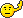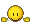Author:  Cassandra [ Sat Jan 09, 2021 11:44 am ]
Post subject:  KataGo @ Igo Hatsuyoron 120 => "very early" guzumi

`[go]\$\$Bcm31\$\$ +---------------------------------------+\$\$ | . . . . . . . . . . . . . . . . O X . |\$\$ | . . . . . . . X O O . X O . . . . O . |\$\$ | . . . . . X . O . . . X . . . . O . . |\$\$ | . X O , . . . . . O . X . O O , . . . |\$\$ | . . X X . X . . . . O X X . X X O O . |\$\$ | . X . O . O . . . . . . X . X O . a . |\$\$ | . O O O . . O O . . O O . O X . . 1 . |\$\$ | O . . X . . . . . . . . . . X X X X X |\$\$ | . . . X . . . O . . O . X X X X . O O |\$\$ | X O O , . X . . . O . X X O O O O . . |\$\$ | X . . O X . . . . O . X O O O . X X X |\$\$ | X . . O X . . . X O . X O O X X X O O |\$\$ | X X . . X . . O c O . X O . O . X X O |\$\$ | . O O X O X . . . O . X X O O O X O O |\$\$ | . . X . . X . . . . X . O X X X O O O |\$\$ | O X X , X . . O . , O O O X O X O O X |\$\$ | . . . . O X . . . . 2 Y Y . O X X O X |\$\$ | O . X . . . b . O . O O O X O X . O X |\$\$ | . X . . . . . . . . . . O X X X . O . |\$\$ +---------------------------------------+[/go]`

Black can play the guzumi earliest with move 31, just because the length of the hanezeki's tail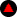has to be at least two stones to enable a tenuki from the bottom.

As a matter of course, White could answer locally at a as usual, reverting to the known solution.

However, according to the new KataGo network, she might also consider blocking the hanezeki's tail with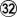at the bottom. After this block the game will also end with two points for White (komi = 1).

Jumping to b, instead, threatens Black's lower left corner, and secures life for White's bottom group. The further path of the hanezeki's tail will be stopped with White c latest. However, according to our trials, this alternative line will be one point worse for White.

For your interest, I add an example of the ensuing play on the board.
(Disclaimer: my laptop is quite weak, so please take the SGF with a grain of salt.)

EDIT: The "Date" shown in the SGF is not correct, had not been updated...

 Attachments:KataGoNew_GuzumiMove31.sgf [4.84 KiB] Downloaded 794 times

 Author: johnsmith [ Sat Jan 09, 2021 1:21 pm ] Post subject: Re: Igo Hatsuyoron 120 => new specific KataGo network in dev Cassandra wrote:Starting from the problem's starting position, it evaluated Black's win rate for komi = -0.5 / -1 / -1.5 with (about) 5% / 50% / 95 %, which should be sufficient evidence that White will really win the game by two points at a komi of 1 point! And that the problem does not have any flaw!I assume the original komi of 1 in the starting position is due to white taking a black stone at t6. Thus the komi of 1 to accommodate that one stone, making the starting count of black and white stones of both 71. Though I like your idea here igohatsuyoron120.de wrote:The original of Igo Hatsuyoron does not give any aims to achieve, but has either "Black to play" or "White to play" only. There is another possible explanation for the fact that there are 71 White, but only 70 Black stones on the board – leaving aside the capture of one Black stone before the problem's setup: It was White – and not Black– who initiated the game.How did that go under radar for so many yearsSo, in that case:black to play, starting stones: black 71, white 71. komi=1 => w+2 (komi of 1 because of the captured black stone)black to play, starting stones: black 70, white 71. komi=0 => w+1Did I get this right? How did you get jigo?

 Author: Cassandra [ Sat Jan 09, 2021 1:48 pm ] Post subject: Re: Igo Hatsuyoron 120 => new specific KataGo network in dev johnsmith wrote:So, in that case:black to play, starting stones: black 71, white 71. komi=1 => w+2 (komi of 1 because of the captured black stone)black to play, starting stones: black 70, white 71. komi=0 => w+1Did I get this right?Yes, you are right.Quote:How did you get jigo?Neural networks in Go work best, in the case they encounter a game as close as possible.Therefore, even if we assume a White win by one point with komi = 0, the game ends not as close as possible.To achieve this aim, we have to set komi = -1, which will turn the outcome of the game to jigo. This corresponds with Black's assumed win rate of 50% in the very beginning of the solution sequence.Let's assume that komi is set to -0.5, what will happen?It is clear that Black will be unable to win the game (remember the assumed 5% win rate for Black in this case), but the AI player who has the Black stones will tend to choose somewhat "risky" moves, intending to create some complications that might enable him to turn the tables.As a result, it is not unlikely that White will win such game by more than only 0.5 points (due to "objective" mistakes that Black made).Another example:If you wanted the AI to provide you with the best counter for White after a Black mistake (this will also be true for a "real" game), you should reduce the komi (seen from White's point of view) according to the (probable) value of Black's mistake.After this adjustment of the komi, the game is "close" again, and White will do as best as she can.

 Author: Friday9i [ Sat Jan 09, 2021 3:20 pm ] Post subject: Re: KataGo @ Igo Hatsuyoron 120 Just a precision regarding the game from Cassandra above:After the "very early guzumi" at move 31, White can simply answer with W S14, which reverts to the standard solution.As shown by the game provided by Cassandra, a (riskier) possible continuation for W is L3.But there are alternative moves: White F2 or White G2 (at move 32) are possible, with comparable winrates (~30% wr like with L3, vs ~50% wr for the standard S14 answer) and apparently identical results, if we believe the net and the thousands of selfplay games played from these starting points.But these lines are still not 100% clear, opinion of the nets may well evolve in the coming weeks, we'll see.

 Author: johnsmith [ Sat Jan 09, 2021 3:30 pm ] Post subject: Re: KataGo @ Igo Hatsuyoron 120 Thanks for the explanation. So you intentionally set the komi to -1 to find the best possible moves there are. That makes sense.I wonder if Inoue Dōsetsu Inseki had deliberately set the stones for the big sacrifice to trick us into a variation without a solution (by 1 point heh), but he inteded the early gozumi all along.

 Author: Cassandra [ Sat Jan 09, 2021 5:08 pm ] Post subject: Re: KataGo @ Igo Hatsuyoron 120 johnsmith wrote:I wonder if Inoue Dōsetsu Inseki had deliberately set the stones for the big sacrifice to trick us into a variation without a solution (by 1 point heh), but he inteded the early gozumi all along.If ever, I would like to assume that it is just the other way round.With the very early guzumi, there are too many roads to Rome for a human player, whilst the known solution is a one-way-street (changes in the order of moves not considered).No two games after the very early guzumi were the same on my laptop. It seems that there are always several miai options, at every stage of the game. The handling of the area around the nakade, of the entire centre, and to top left corner can be completely different from game to game.EDIT: If W 32 at G2 was feasible to produce a White win by two points (komi = 1), then this would be the also true with a length of three, and four, stones of the tail (for the moment of Black's guzumi).Probably, this alternative line found by Friday9i was unavoidable, a kind of side effect due to the middle-game starting position of the problem.Apparently, our very early evaluation of this very early guzumi (can be found in the section "Timing of the Guzumi" on our website) was mistaken (it favoured White too much), mainly because our weakness in the endgame (and our missing knowledge of some of KataGo's strategies for Black). But let us meet about halfway and assume that we had calculated a White win with 6 points after Black's guzumi with move 31. The growing tail of the hanezeki destroys potential White territory in the centre; the larger it becomes, the more destructive it is (until a certain point). Even in that case, there would be a(nother) moment in the game, when an early guzumi with White tenuki would have had the same outcome as the currently known solution.It might be due to the genius of Inoue Dôsetsu Inseki that this moment is the earliest possible one, as it seems now.

 Author: johnsmith [ Sun Jan 10, 2021 3:47 am ] Post subject: Re: KataGo @ Igo Hatsuyoron 120 Cassandra wrote:johnsmith wrote:I wonder if Inoue Dōsetsu Inseki had deliberately set the stones for the big sacrifice to trick us into a variation without a solution (by 1 point heh), but he inteded the early gozumi all along.If ever, I would like to assume that it is just the other way round.Agreed, it's just more beautiful that way. Can't wait for the new discoveries.

 Author: Cassandra [ Sun Jan 10, 2021 5:44 am ] Post subject: Igo Hatsuyoron 120 and KataGo new Guzumi delayed too long => White's surprising wedge in the left centre Click Here To Show Diagram Code`[go]\$\$Bcm93\$\$ +---------------------------------------+\$\$ | . . . . . . . . . . . . X . . . O X . |\$\$ | . . . . . . . X O O . X O X O . . O . |\$\$ | . . . . . X . O . . . X O . . O O . . |\$\$ | . X O , . . . . . O . X . O O X . . . |\$\$ | . . X X X X O O O . O X X . X X O O . |\$\$ | . X . O O O X X X O . . X . X O . . . |\$\$ | O O O O . X O O X X O O . O X . . 2 . |\$\$ | O X . X . X . O O X O . . . X X X X X |\$\$ | O X . X . . . O X X O . X X X X . O O |\$\$ | X O O X . X . O X O O X X O O O O . . |\$\$ | X X O O X . . O X O . X O O O . X X X |\$\$ | X X . O X . . O X O . X O O X X X O O |\$\$ | X X O O X . . O X O . X O . O . X X O |\$\$ | X O O . O X . O X O . X X O O O X O O |\$\$ | X O X O . X . O X O X . O X X X O O O |\$\$ | O X X 1 X . . O X O O O O X O X O O X |\$\$ | O . . . O X . O X X X X X . O X X O X |\$\$ | O . X . . . X O O O O O O X O X . O X |\$\$ | . X . . . . . O . . . . O X X X . O . |\$\$ +---------------------------------------+[/go]`The old KataGo net was fine with delaying the guzumi even until after the Nakade Sequence at the left.However, our investigation showed that -- after Black's final move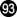in the lower left -- White will occupy the guzumi point herself with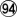. Click Here To Show Diagram Code`[go]\$\$Bcm95\$\$ +---------------------------------------+\$\$ | . . . . . . . . . . . . X . . . O X . |\$\$ | . . . . . . b X O O . X O X O . . O . |\$\$ | . . . . . X . O . . . X O a 2 O O . . |\$\$ | . X O , . . . . . O . X . O O X . . . |\$\$ | . . X X X X O O O . O X X 1 X X O O . |\$\$ | . X . O O O X X X O . . X . X O . . . |\$\$ | O O O O . X O O X X O O . O X . . O . |\$\$ | O X . X . X . O O X O . . . X X X X X |\$\$ | O X . X . . . O X X O . X X X X . O O |\$\$ | X O O X . X . O X O O X X O O O O . . |\$\$ | X X O O X . . O X O . X O O O . X X X |\$\$ | X X . O X . . O X O . X O O X X X O O |\$\$ | X X O O X . . O X O . X O . O . X X O |\$\$ | X O O . O X . O X O . X X O O O X O O |\$\$ | X O X O . X . O X O X . O X X X O O O |\$\$ | O X X X X . . O X O O O O X O X O O X |\$\$ | O . . . O X . O X X X X X . O X X O X |\$\$ | O . X . . . X O O O O O O X O X . O X |\$\$ | . X . . . . . O . . . . O X X X . O . |\$\$ +---------------------------------------+[/go]`As usual with the "classical" line of play (i.e. without Black's guzumi), it is best for Black to play Michael Redmond's kikashi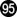in the bamboo joint just now.White is forced to answer with the solid connection.If she chose the atari at a, instead, she would have lost a tempo in the Main Semeai, enabling Black to take the large endgame point of b in the upper left corner, so winning the game. Click Here To Show Diagram Code`[go]\$\$Bcm97\$\$ +---------------------------------------+\$\$ | . . . . . . . . . . . . X . . . O X . |\$\$ | . . . . . . . X O O . X O X O . . O . |\$\$ | . . . . . X . O . . . X O . O O O . . |\$\$ | . X O , . . . . . O . X . O O X . . . |\$\$ | . . X X X X O O O . O X X X X X O O . |\$\$ | . X . O O O X X X O . . X . X O . . . |\$\$ | O O O O a X O O X X O O . O X . 2 O . |\$\$ | O X . X . X . O O X O . . . X X X X X |\$\$ | O X . X . . . O X X O . X X X X . O O |\$\$ | X O O X . X . O X O O X X O O O O . . |\$\$ | X X O O X . . O X O . X O O O . X X X |\$\$ | X X . O X . . O X O . X O O X X X O O |\$\$ | X X O O X . . O X O . X O . O . X X O |\$\$ | X O O 3 O X . O X O . X X O O O X O O |\$\$ | X O X O 1 X . O X O X . O X X X O O O |\$\$ | O X X X X . . O X O O O O X O X O O X |\$\$ | O . . . O X . O X X X X X . O X X O X |\$\$ | O . X . . . X O O O O O O X O X . O X |\$\$ | . X . . . . . O . . . . O X X X . O . |\$\$ +---------------------------------------+[/go]`Black will give atari at White's two stones in the lower left, in order to strenghten his position in the left centre.White avoids some complications in the upper right (to be explained in some later posting) by defending there with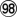first.Black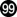captures White's two stones.Blocking at a in the left centre, instead, will result in the same final score, as White will be able to connect her two stones later, or to gain alternatively in the forthcoming endgame on the left side of the board. Click Here To Show Diagram Code`[go]\$\$Wcm100\$\$ +---------------------------------------+\$\$ | . . . 8 5 . . . . . . . X . . . O X . |\$\$ | . . . 6 2 1 7 X O O . X O X O . . O . |\$\$ | . . . 4 3 X . O . . . X O . O O O . . |\$\$ | . X O , . . . . . O . X . O O X . . . |\$\$ | . . X X X X O O O . O X X X X X O O . |\$\$ | . X . O O O X X X O . . X . X O . . . |\$\$ | O O O O . X O O X X O O . O X . O O . |\$\$ | O X . X . X . O O X O . . . X X X X X |\$\$ | O X . X . . . O X X O . X X X X . O O |\$\$ | X O O X . X . O X O O X X O O O O . . |\$\$ | X X O O X . . O X O . X O O O . X X X |\$\$ | X X . O X . . O X O . X O O X X X O O |\$\$ | X X O O X . . O X O . X O . O . X X O |\$\$ | X O O X . X . O X O . X X O O O X O O |\$\$ | X O X . X X . O X O X . O X X X O O O |\$\$ | O X X X X . . O X O O O O X O X O O X |\$\$ | O . . . O X . O X X X X X . O X X O X |\$\$ | O . X . . . X O O O O O O X O X . O X |\$\$ | . X . . . . . O . . . . O X X X . O . |\$\$ +---------------------------------------+[/go]`Black is still par with the Punishment Semeai Liberty Level, so White starts the endgame on the left side "early". Click Here To Show Diagram Code`[go]\$\$Wcm108\$\$ +---------------------------------------+\$\$ | . . . X O . . . . . a . X . . . O X . |\$\$ | . . . X X O O X O O . X O X O . . O . |\$\$ | . . . X O X . O . . . X O . O O O . . |\$\$ | . X O , . . 6 . . O . X . O O X . . . |\$\$ | . . X X X X O O O . O X X X X X O O . |\$\$ | . X . O O O X X X O . . X . X O . . . |\$\$ | O O O O b X O O X X O O . O X . O O . |\$\$ | O X . X c X . O O X O . . . X X X X X |\$\$ | O X . X . 9 . O X X O . X X X X . O O |\$\$ | X O O X . X . O X O O X X O O O O . . |\$\$ | X X O O X . . O X O . X O O O . X X X |\$\$ | X X . O X . . O X O . X O O X X X O O |\$\$ | X X O O X . . O X O . X O . O . X X O |\$\$ | X O O X . X . O X O . X X O O O X O O |\$\$ | X O X . X X . O X O X . O X X X O O O |\$\$ | O X X X X 8 . O X O O O O X O X O O X |\$\$ | O . . . O X 7 O X X X X X . O X X O X |\$\$ | O . X . 2 1 X O O O O O O X O X . O X |\$\$ | . X . . 4 3 5 O . . . . O X X X . O . |\$\$ +---------------------------------------+[/go]`Black plays tenuki with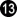, in order to occupy the large endgame point at the top.After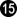, we continued with the Main Semeai with White's peep at a at the upper edge, finally scoring a White win by THREE points (komi = 1), which is one point better for her than the known solution to the problem.KataGo's new net, howewer, found that Black's earlier mistake with not playing the guzumi should be punished even harder.White will play the wedge at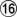in the left centre.Playing KataGo's bad-shape move of b just now would be a mistake.Black already captured White's two stones in the lower left, so he is able to immediately block at c without endangering his position in the left centre. Click Here To Show Diagram Code`[go]\$\$Bcm117\$\$ +---------------------------------------+\$\$ | . . . X O . . . . . . . X . . . O X . |\$\$ | . . . X X O O X O O . X O X O . . O . |\$\$ | . . . X O X . O . . . X O . O O O . . |\$\$ | . X O , . . X . . O . X . O O X . . . |\$\$ | . . X X X X O O O . O X X X X X O O . |\$\$ | . X . O O O X X X O . . X . X O . . . |\$\$ | O O O O . X O O X X O O . O X . O O . |\$\$ | O X . X . X . O O X O . . . X X X X X |\$\$ | O X . X 3 O 1 O X X O . X X X X . O O |\$\$ | X O O X . X 2 O X O O X X O O O O . . |\$\$ | X X O O X . . O X O . X O O O . X X X |\$\$ | X X . O X . . O X O . X O O X X X O O |\$\$ | X X O O X . . O X O . X O . O . X X O |\$\$ | X O O X . X . O X O . X X O O O X O O |\$\$ | X O X . X X . O X O X . O X X X O O O |\$\$ | O X X X X X . O X O O O O X O X O O X |\$\$ | O . . . O X O O X X X X X . O X X O X |\$\$ | O . X . X O . O O O O O O X O X . O X |\$\$ | . X . . X O O O . . . . O X X X . O . |\$\$ +---------------------------------------+[/go]`Black must give atari from the outside. Click Here To Show Diagram Code`[go]\$\$Wcm120\$\$ +---------------------------------------+\$\$ | . . . X O . . . . . 5 6 X . . . O X . |\$\$ | . . . X X O O X O O . X O X O . . O . |\$\$ | . . . X O X . O . . . X O . O O O . . |\$\$ | . X O , . . X . . O . X . O O X . . . |\$\$ | . . X X X X O O O . O X X X X X O O . |\$\$ | . X . O O O X X X O . . X . X O . . . |\$\$ | O O O O 3 X O O X X O O . O X . O O . |\$\$ | O X x X x X 1 O O X O . . . X X X X X |\$\$ | O X x X X 2 X O X X O . X X X X . O O |\$\$ | X O O X a X O O X O O X X O O O O . . |\$\$ | X X O O X 4 . O X O . X O O O . X X X |\$\$ | X X . O X b . O X O . X O O X X X O O |\$\$ | X X O O X c d O X O . X O . O . X X O |\$\$ | X O O X . X . O X O . X X O O O X O O |\$\$ | X O X . X X . O X O X . O X X X O O O |\$\$ | O X X X X X . O X O O O O X O X O O X |\$\$ | O . . . O X O O X X X X X . O X X O X |\$\$ | O . X . X O . O O O O O O X O X . O X |\$\$ | . X . . X O O O . . . . O X X X . O . |\$\$ +---------------------------------------+[/go]`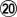is sente.After Black connected at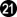, the time is ripe for KataGo's bad-shape move with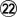.Black has to defend his cutting point at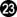-- sooner or later.Please note that White's bad-shape move did not lose any tempo in the Main Semeai, but Black got only TWO points of territory in the left centre (one prisoner included), instead of the "usual" three.If Black does without this defensive move, White would gain by the sequence of W 123, B a, W b, B c, W d in the left centre, once Black had occupied the x liberties of her left-hand group in the Main Semeai.As a result of White's newly found tesuji in the left centre, she will win the game by FOUR points now (komi = 1).

 Author: Cassandra [ Sun Jan 10, 2021 2:39 pm ] Post subject: Re: KataGo @ Igo Hatsuyoron 120 "Classical" way of play => White's weakness in the upper rightWe will still examine the "classical" way of play, i.e. the situation without Black's guzumi in the upper right. Click Here To Show Diagram Code`[go]\$\$Wcm98\$\$ +---------------------------------------+\$\$ | . . . . . 6 . 7 . . . . X . . . O X . |\$\$ | . . . . 2 1 3 X O O . X O X O . . O . |\$\$ | . . . 8 5 X 4 O . . . X O . O O O . . |\$\$ | . X O , . . . . . O . X . O O X . . . |\$\$ | . . X X X X O O O . O X X X X X O O . |\$\$ | . X . O O O X X X O . . X . X O . . . |\$\$ | O O O O . X O O X X O O . O X . a O . |\$\$ | O X . X . X . O O X O . . . X X X X X |\$\$ | O X . X . . . O X X O . X X X X . O O |\$\$ | X O O X . X . O X O O X X O O O O . . |\$\$ | X X O O X . . O X O . X O O O . X X X |\$\$ | X X . O X . . O X O . X O O X X X O O |\$\$ | X X O O X . . O X O . X O . O . X X O |\$\$ | X O O . O X . O X O . X X O O O X O O |\$\$ | X O X O X X . O X O X . O X X X O O O |\$\$ | O X X X X . . O X O O O O X O X O O X |\$\$ | O . . . O X . O X X X X X . O X X O X |\$\$ | O . X . . . X O O O O O O X O X . O X |\$\$ | . X . . . . . O . . . . O X X X . O . |\$\$ +---------------------------------------+[/go]`In order to set the scene for another finding of the new KataGo net, let us assume that White chooses the original line of Yamada Shinji's tsuke in the upper right corner.Please note that White has NOT defended with a in the upper right before starting the endgame at the left side. Click Here To Show Diagram Code`[go]\$\$Wcm106\$\$ +---------------------------------------+\$\$ | . . . . . X . O . . . . X . . . O X . |\$\$ | . . . . X O O . O O . X O X O . . O . |\$\$ | . . . X O X X O . . . X O . O O O . . |\$\$ | . X O , 8 . 6 7 . O . X . O O X . . . |\$\$ | . . X X X X O O O . O X X X X X O O . |\$\$ | . X . O O O X X X O . . X . X O . . . |\$\$ | O O O O . X O O X X O O . O X . . O . |\$\$ | O X . X . X . O O X O . . . X X X X X |\$\$ | O X . X . . . O X X O . X X X X . O O |\$\$ | X O O X . X . O X O O X X O O O O . . |\$\$ | X X O O X . . O X O . X O O O . X X X |\$\$ | X X . O X . . O X O . X O O X X X O O |\$\$ | X X O O X . . O X O . X O . O . X X O |\$\$ | X O O . O X . O X O . X X O O O X O O |\$\$ | X O X O X X . O X O X . O X X X O O O |\$\$ | O X X X X 0 . O X O O O O X O X O O X |\$\$ | O . . . O X 9 O X X X X X . O X X O X |\$\$ | O . X . 2 1 X O O O O O O X O X . O X |\$\$ | . X . . 4 3 5 O . . . . O X X X . O . |\$\$ +---------------------------------------+[/go]`The "early" endgame continues in the lower left corner.Again, Black plays tenuki with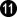, in order to play a profitable move at the top. Click Here To Show Diagram Code`[go]\$\$Wcm116\$\$ +---------------------------------------+\$\$ | . . . . . X . O . . . . X . . . O X . |\$\$ | . . . . X O O . O O . X O X O . . O . |\$\$ | . . . X . X X O . . . X O . O O O . . |\$\$ | . X O , X . X O . O . X . O O X . . . |\$\$ | . . X X X X O O O . O X X X X X O O . |\$\$ | . X . O O O X X X O . . X . X O 3 9 5 |\$\$ | O O O O 6 X O O X X O O . O X . 2 O 4 |\$\$ | O X . X . X . O O X O . . . X X X X X |\$\$ | O X . X . 8 . O X X O . X X X X . O O |\$\$ | X O O X . X 7 O X O O X X O O O O . . |\$\$ | X X O O X . . O X O . X O O O . X X X |\$\$ | X X . O X . . O X O . X O O X X X O O |\$\$ | X X O O X . . O X O . X O . O . X X O |\$\$ | X O O 1 O X . O X O . X X O O O X O O |\$\$ | X O X O X X . O X O X . O X X X O O O |\$\$ | O X X X X X . O X O O O O X O X O O X |\$\$ | O . . . O X O O X X X X X . O X X O X |\$\$ | O . X . X O . O O O O O O X O X . O X |\$\$ | . X . . X O O O . . . . O X X X . O . |\$\$ +---------------------------------------+[/go]`Black ended in gote at the top, so White will connect her two stones in the lower left with, gaining two points overall.However, she lost two tempi in the Main Semeai by this connection.This makes Black's push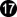in the guzumi area possible, which will result in a gain of TWO points overall for Black.Here, White blocks with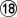, but she could simplify the issue by connecting at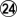immediately, instead.Thereafter, Black plays another push into White's potential territory with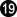. Black could have changed the order of moves here, and started at the edge.White blocks again with.Again, immediately connecting atcould simplify the matter.At first sight, it seems that Black lost two tempi in the Main Semeai by his new procedure (White's moves occupied liberties of his large group at the right) -- making him a victim of the Punishment Semeai -- but this is not true.Sooner or later, White will have to connect at, also losing one tempo in the Main Semeai.White lost three points of territory here, Black lost one tempo in the Main Semeai effectively (two minus one for White), so the net effect is a loss of TWO points for White (in the Capture Variation, which will be better for White than the Semeai Variation).This means that White must be careful to not provide Black with a single additional Main Semeai Liberty, if she had not yet defended her potential territory in the upper right. Click Here To Show Diagram Code`[go]\$\$Wcm124\$\$ +---------------------------------------+\$\$ | . . . . . X . O . . 1 2 X . . . O X . |\$\$ | . . . . X O O . O O . X O X O . . O . |\$\$ | . . . X . X X O . . . X O . O O O . . |\$\$ | . X O , X . X O . O . X . O O X . . . |\$\$ | . . X X X X O O O . O X X X X X O O a |\$\$ | . X . O O O X X X O . . X . X O O 4 O |\$\$ | O O O O X X O O X X O O 3 O X . X O X |\$\$ | O X . X . X . O O X O . . . X X X X X |\$\$ | O X . X . X . O X X O . X X X X . O O |\$\$ | X O O X . X O O X O O X X O O O O . . |\$\$ | X X O O X . . O X O . X O O O . X X X |\$\$ | X X . O X . . O X O . X O O X X X O O |\$\$ | X X O O X . . O X O . X O . O . X X O |\$\$ | X O O O O X . O X O . X X O O O X O O |\$\$ | X O X O X X . O X O X . O X X X O O O |\$\$ | O X X X X X . O X O O O O X O X O O X |\$\$ | O . . . O X O O X X X X X . O X X O X |\$\$ | O . X . X O X O O O O O O X O X . O X |\$\$ | . X . . X O O O . . . . O X X X . O . |\$\$ +---------------------------------------+[/go]`Let's assume that White does not want to connect the ko shape in the upper right, but continues with the Main Semeai.Black will capture the ko with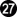.White is forced to fight this ko, because simply connecting at a would cost her another point.However, White does not have any change to "win" this ko fight (i.e. to leave the ko shape open), because Black can use his liberty-occupying moves of the Main Semeai as ko threats (contrary to White, who has to choose "real" threats).This implies that White has to connect the ko lastest after she had invested her very last "real" ko threat.It would be much simpler to do so in the very beginning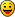Author: Cassandra [ Mon Jan 11, 2021 4:05 am ] Post subject: Re: KataGo @ Igo Hatsuyoron 120 "Classical" way of play => Black's weakness in the upper leftSEATBELTS FASTENED?OK, then let's go...We still examine the "classical" way of play, i.e. the situation without Black's guzumi in the upper right.Please note that I have not checked yet whether the following variations, concerning the upper left corner only, are also valid with the Guzumi Exchange played. Click Here To Show Diagram Code`[go]\$\$Wcm108\$\$ +---------------------------------------+\$\$ | . . . . . B . W . . . . X . . . O X . |\$\$ | . . . . B W W . O O . X O X O . . O . |\$\$ | . . . B W X B O . . . X O . O O O . . |\$\$ | . X O , . . 1 . . O . X . O O X . . . |\$\$ | . . X X X X O O O . O X X X X X O O . |\$\$ | . X . O O O X X X O . . X . X O . . . |\$\$ | O O O O . X O O X X O O . O X . Q O . |\$\$ | O X . X . X . O O X O . . . X X X X X |\$\$ | O X . X . . . O X X O . X X X X . O O |\$\$ | X O O X . X . O X O O X X O O O O . . |\$\$ | X X O O X . . O X O . X O O O . X X X |\$\$ | X X . O X . . O X O . X O O X X X O O |\$\$ | X X O O X . . O X O . X O . O . X X O |\$\$ | X O O Y . X . O X O . X X O O O X O O |\$\$ | X O X . X X . O X O X . O X X X O O O |\$\$ | O X X X X . . O X O O O O X O X O O X |\$\$ | O . . . O X . O X X X X X . O X X O X |\$\$ | O . X . . a X O O O O O O X O X . O X |\$\$ | . X . . . . . O . . . . O X X X . O . |\$\$ +---------------------------------------+[/go]`After the classical line of play after Yamada Shinji's tsuke in the upper left (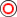,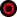), White does NOT continue the endgame with a move at a in the lower left corner here, but gives atari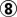in the upper left immediately.For the sake of simplicity, let's assume that the exchange of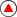forhas already been inserted. Click Here To Show Diagram Code`[go]\$\$Wcm108\$\$ +---------------------------------------+\$\$ | . . . . . X . O . . . . X . . . O X . |\$\$ | . . . . X O O . O O . X O X O . . O . |\$\$ | . . . X O X X O . . . X O . O O O . . |\$\$ | . X O , 2 a O . . O . X . O O X . . . |\$\$ | . . X X X X O O O . O X X X X X O O . |\$\$ | . X . O O O X X X O . . X . X O . . . |\$\$ | O O O O . X O O X X O O . O X . O O . |\$\$ | O X . X . X . O O X O . . . X X X X X |\$\$ | O X . X . . . O X X O . X X X X . O O |\$\$ | X O O X . X . O X O O X X O O O O . . |\$\$ | X X O O X . . O X O . X O O O . X X X |\$\$ | X X . O X . . O X O . X O O X X X O O |\$\$ | X X O O X . . O X O . X O . O . X X O |\$\$ | X O O X . X . O X O . X X O O O X O O |\$\$ | X O X . X X . O X O X . O X X X O O O |\$\$ | O X X X X . . O X O O O O X O X O O X |\$\$ | O . . . O X . O X X X X X . O X X O X |\$\$ | O . X . . . X O O O O O O X O X . O X |\$\$ | . X . . . . . O . . . . O X X X . O . |\$\$ +---------------------------------------+[/go]`Black has to capture with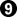.Intending to maximise his corner territory by connecting at a would be mistaken, see the next posting. Click Here To Show Diagram Code`[go]\$\$Wcm110\$\$ +---------------------------------------+\$\$ | . . . . . X . O . . . . X . . . O X . |\$\$ | . . . . X O O . O O . X O X O . . O . |\$\$ | . . . X 8 X X O . . . X O . O O O . . |\$\$ | . X O , X 7 O . . O . X . O O X . . . |\$\$ | . . X X X X O O O . O X X X X X O O . |\$\$ | . X . O O O X X X O . . X . X O . . . |\$\$ | O O O O . X O O X X O O . O X . O O . |\$\$ | O X . X . X . O O X O . . . X X X X X |\$\$ | O X . X . . . O X X O . X X X X . O O |\$\$ | X O O X . X . O X O O X X O O O O . . |\$\$ | X X O O X . . O X O . X O O O . X X X |\$\$ | X X . O X . . O X O . X O O X X X O O |\$\$ | X X O O X . . O X O . X O . O . X X O |\$\$ | X O O X . X . O X O . X X O O O X O O |\$\$ | X O X . X X . O X O X . O X X X O O O |\$\$ | O X X X X . . O X O O O O X O X O O X |\$\$ | O . . . O X 6 O X X X X X . O X X O X |\$\$ | O . X . 2 1 X O O O O O O X O X . O X |\$\$ | . X . . 4 3 5 O . . . . O X X X . O . |\$\$ +---------------------------------------+[/go]`After having gained in the upper left, White continues with the usual endgame sequence in the lower left.There is no other large endgame point remaining on the board, so Black will connect his single stone with.Please note that Black is still par with the Punishment Semeai Liberty Level, so White will give atariafter her decision point; Black will connect at. Click Here To Show Diagram Code`[go]\$\$Wc\$\$ +---------------------------------------+\$\$ | . . . . . X . O . . . . X . . . O X . |\$\$ | . . . . X O O . O O . X O X O . . O . |\$\$ | . . . X Z X X O . . . X O . O O O . . |\$\$ | . X O , X P O T . O . X . O O X . . . |\$\$ | . . X X X X O O O . O X X X X X O O . |\$\$ | . X . O O O X X X O . . X . X O . . . |\$\$ | O O O O . X O O X X O O . O X . O O . |\$\$ | O X . X . X . O O X O . . . X X X X X |\$\$ | O X . X . a . O X X O . X X X X . O O |\$\$ | X O O X . X . O X O O X X O O O O . . |\$\$ | X X O O X . . O X O . X O O O . X X X |\$\$ | X X . O X . . O X O . X O O X X X O O |\$\$ | X X O O X . . O X O . X O . O . X X O |\$\$ | X O O X . X . O X O . X X O O O X O O |\$\$ | X O X . X X . O X O X . O X X X O O O |\$\$ | O X X X X . . O X O O O O X O X O O X |\$\$ | O . . . O X X O X X X X X . O X X O X |\$\$ | O . X . X O # O O O O O O X O X . O X |\$\$ | . X . . X O O O . . . . O X X X . O . |\$\$ +---------------------------------------+[/go]`Compared with the "classical" line of play ...-- Black lost two points of territory in the upper left (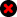,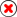).-- White gained one point of territory there (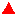).-- Black connected his single stone in the lower left (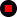), so gaining two points.In total, White gained ONE point.Please note that she will gain another point by choosing the newly discovered wedge at a in the left centre.The game will now end with a White win by TWO points, instead of becoming a Jigo.

 Author: Cassandra [ Mon Jan 11, 2021 6:21 am ] Post subject: Re: KataGo @ Igo Hatsuyoron 120 "Classical" way of play => Black's weakness in the upper left (contd.) Click Here To Show Diagram Code`[go]\$\$Wcm108\$\$ +---------------------------------------+\$\$ | . . . . . X . O . . . . X . . . O X . |\$\$ | . . . . X O O . O O . X O X O . . O . |\$\$ | . . . X O X X O . . . X O . O O O . . |\$\$ | . X O , . 2 O . . O . X . O O X . . . |\$\$ | . . X X X X O O O . O X X X X X O O . |\$\$ | . X . O O O X X X O . . X . X O . . . |\$\$ | O O O O . X O O X X O O . O X . O O . |\$\$ | O X . X . X . O O X O . . . X X X X X |\$\$ | O X . X . . . O X X O . X X X X . O O |\$\$ | X O O X . X . O X O O X X O O O O . . |\$\$ | X X O O X . . O X O . X O O O . X X X |\$\$ | X X . O X . . O X O . X O O X X X O O |\$\$ | X X O O X . . O X O . X O . O . X X O |\$\$ | X O O X . X . O X O . X X O O O X O O |\$\$ | X O X . X X . O X O X . O X X X O O O |\$\$ | O X X X X . . O X O O O O X O X O O X |\$\$ | O . . . O X . O X X X X X . O X X O X |\$\$ | O . X . . . X O O O O O O X O X . O X |\$\$ | . X . . . . . O . . . . O X X X . O . |\$\$ +---------------------------------------+[/go]`Black is too greedy, when he closes the right-hand hole in his corner's encirclement. Click Here To Show Diagram Code`[go]\$\$Wcm108\$\$ +---------------------------------------+\$\$ | . . . . . X . O . . . . X . . . O X . |\$\$ | . . . . X O O . O O . X O X O . . O . |\$\$ | . . . X O X X O . . . X O . O O O . . |\$\$ | 3 X O , . X O . . O . X . O O X . . . |\$\$ | . . X X X X O O O . O X X X X X O O . |\$\$ | . X . O O O X X X O . . X . X O . . . |\$\$ | O O O O . X O O X X O O . O X . O O . |\$\$ | O X . X . X . O O X O . . . X X X X X |\$\$ | O X . X . . . O X X O . X X X X . O O |\$\$ | X O O X . X . O X O O X X O O O O . . |\$\$ | X X O O X . . O X O . X O O O . X X X |\$\$ | X X . O X . . O X O . X O O X X X O O |\$\$ | X X O O X . . O X O . X O . O . X X O |\$\$ | X O O X . X . O X O . X X O O O X O O |\$\$ | X O X . X X . O X O X . O X X X O O O |\$\$ | O X X X X . . O X O O O O X O X O O X |\$\$ | O . . . O X . O X X X X X . O X X O X |\$\$ | O . X . . . X O O O O O O X O X . O X |\$\$ | . X . . . . . O . . . . O X X X . O . |\$\$ +---------------------------------------+[/go]`It made "boooooooom".White's torpedo hit Black's vessel below the waterline.How does Black intend to stop the impending water ingress?

 Author: Cassandra [ Mon Jan 11, 2021 8:36 am ] Post subject: Re: KataGo @ Igo Hatsuyoron 120 "Classical" way of play => Black's weakness in the upper left (contd.)Black has to passively back away. Click Here To Show Diagram Code`[go]\$\$Wcm108\$\$ +---------------------------------------+\$\$ | . . . . . X . O . . . . X . . . O X . |\$\$ | . . . . X O O . O O . X O X O . . O . |\$\$ | b 4 . X O X X O . . . X O . O O O . . |\$\$ | O X O , . X O . . O . X . O O X . . . |\$\$ | c a X X X X O O O . O X X X X X O O . |\$\$ | d X . O O O X X X O . . X . X O . . . |\$\$ | O O O O . X O O X X O O . O X . O O . |\$\$ | O X . X . X . O O X O . . . X X X X X |\$\$ | O X . X . . . O X X O . X X X X . O O |\$\$ | X O O X . X . O X O O X X O O O O . . |\$\$ | X X O O X . . O X O . X O O O . X X X |\$\$ | X X . O X . . O X O . X O O X X X O O |\$\$ | X X O O X . . O X O . X O . O . X X O |\$\$ | X O O X . X . O X O . X X O O O X O O |\$\$ | X O X . X X . O X O X . O X X X O O O |\$\$ | O X X X X . . O X O O O O X O X O O X |\$\$ | O . . . O X . O X X X X X . O X X O X |\$\$ | O . X . . . X O O O O O O X O X . O X |\$\$ | . X . . . . . O . . . . O X X X . O . |\$\$ +---------------------------------------+[/go]`Black's best option here to answer White's submarine attack is to passively back away with.Alternatively, he might consider connecting at a, instead, achieving the same final score.Any other move in the corner would be even worse for him, the more aggressive the worse. Click Here To Show Diagram Code`[go]\$\$Wcm112\$\$ +---------------------------------------+\$\$ | . . . . . X . O . . . . X . . . O X . |\$\$ | . . . . X O O . O O . X O X O . . O . |\$\$ | 2 X . X O X X O . . . X O . O O O . . |\$\$ | O X O , . X O . . O . X . O O X . . . |\$\$ | 1 4 X X X X O O O . O X X X X X O O . |\$\$ | 3 X . O O O X X X O . . X . X O . . . |\$\$ | O O O O . X O O X X O O . O X . O O . |\$\$ | O X . X . X . O O X O . . . X X X X X |\$\$ | O X . X . . . O X X O . X X X X . O O |\$\$ | X O O X . X . O X O O X X O O O O . . |\$\$ | X X O O X . . O X O . X O O O . X X X |\$\$ | X X . O X . . O X O . X O O X X X O O |\$\$ | X X O O X . . O X O . X O . O . X X O |\$\$ | X O O X . X . O X O . X X O O O X O O |\$\$ | X O X . X X . O X O X . O X X X O O O |\$\$ | O X X X X . . O X O O O O X O X O O X |\$\$ | O . . . O X . O X X X X X . O X X O X |\$\$ | O . X . . . X O O O O O O X O X . O X |\$\$ | . X . . . . . O . . . . O X X X . O . |\$\$ +---------------------------------------+[/go]`White connects to the outside, at the cost of one tempo in the Main Semeai. Click Here To Show Diagram Code`[go]\$\$Wcm116\$\$ +---------------------------------------+\$\$ | . . . . . X . O . . . . X . . . O X . |\$\$ | . . . . X O O . O O . X O X O . . O . |\$\$ | X X . X O X X O . . . X O . O O O . . |\$\$ | O X O , . X O . . O . X . O O X b c . |\$\$ | O X X X X X O O O . O X X X X X O O . |\$\$ | O X . O O O X X X O . . X . X O . . . |\$\$ | O O O O . X O O X X O O . O X . O O . |\$\$ | O X . X . X . O O X O . . . X X X X X |\$\$ | O X . X . a . O X X O . X X X X . O O |\$\$ | X O O X . X . O X O O X X O O O O . . |\$\$ | X X O O X . . O X O . X O O O . X X X |\$\$ | X X . O X . . O X O . X O O X X X O O |\$\$ | X X O O X . . O X O . X O . O . X X O |\$\$ | X O O X . X . O X O . X X O O O X O O |\$\$ | X O X . X X . O X O X . O X X X O O O |\$\$ | O X X X X . . O X O O O O X O X O O X |\$\$ | O . . . O X 6 O X X X X X . O X X O X |\$\$ | O . X . 2 1 X O O O O O O X O X . O X |\$\$ | . X . . 4 3 5 O . . . . O X X X . O . |\$\$ +---------------------------------------+[/go]`The endgame in the lower left corner follows.connects, as there is nothing move valuable left on the board.White will continue in the left centre with her wedge at a.Black will use his surplus Main Semeai Liberty for reducing White's territory in the upper right by one point, e.g. with B b, W c.White gained three points in the upper left corner, so she will win this game with FOUR points.

 Author: Bill Spight [ Mon Jan 11, 2021 10:00 am ] Post subject: Re: KataGo @ Igo Hatsuyoron 120 Cassandra wrote:"Classical" way of play => White's weakness in the upper rightWe will still examine the "classical" way of play, i.e. the situation without Black's guzumi in the upper right. Click Here To Show Diagram Code`[go]\$\$Wcm98\$\$ +---------------------------------------+\$\$ | . . . . . 6 . 7 . . . . X . . . O X . |\$\$ | . . . . 2 1 3 X O O . X O X O . . O . |\$\$ | . . . 8 5 X 4 O . . . X O . O O O . . |\$\$ | . X O , . . . . . O . X . O O X . . . |\$\$ | . . X X X X O O O . O X X X X X O O . |\$\$ | . X . O O O X X X O . . X . X O . . . |\$\$ | O O O O . X O O X X O O . O X . a O . |\$\$ | O X . X . X . O O X O . . . X X X X X |\$\$ | O X . X . . . O X X O . X X X X . O O |\$\$ | X O O X . X . O X O O X X O O O O . . |\$\$ | X X O O X . . O X O . X O O O . X X X |\$\$ | X X . O X . . O X O . X O O X X X O O |\$\$ | X X O O X . . O X O . X O . O . X X O |\$\$ | X O O . O X . O X O . X X O O O X O O |\$\$ | X O X O X X . O X O X . O X X X O O O |\$\$ | O X X X X . . O X O O O O X O X O O X |\$\$ | O . . . O X . O X X X X X . O X X O X |\$\$ | O . X . . . X O O O O O O X O X . O X |\$\$ | . X . . . . . O . . . . O X X X . O . |\$\$ +---------------------------------------+[/go]`In order to set the scene for another finding of the new KataGo net, let us assume that White chooses the original line of Yamada Shinji's tsuke in the upper right corner.Please note that White has NOT defended with a in the upper right before starting the endgame at the left side. Click Here To Show Diagram Code`[go]\$\$Wcm106\$\$ +---------------------------------------+\$\$ | . . . . . X . O . . . . X . . . O X . |\$\$ | . . . . X O O . O O . X O X O . . O . |\$\$ | . . . X O X X O . . . X O . O O O . . |\$\$ | . X O , 8 . 6 7 . O . X . O O X . . . |\$\$ | . . X X X X O O O . O X X X X X O O . |\$\$ | . X . O O O X X X O . . X . X O . . . |\$\$ | O O O O . X O O X X O O . O X . . O . |\$\$ | O X . X . X . O O X O . . . X X X X X |\$\$ | O X . X . . . O X X O . X X X X . O O |\$\$ | X O O X . X . O X O O X X O O O O . . |\$\$ | X X O O X . . O X O . X O O O . X X X |\$\$ | X X . O X . . O X O . X O O X X X O O |\$\$ | X X O O X . . O X O . X O . O . X X O |\$\$ | X O O . O X . O X O . X X O O O X O O |\$\$ | X O X O X X . O X O X . O X X X O O O |\$\$ | O X X X X 0 . O X O O O O X O X O O X |\$\$ | O . . . O X 9 O X X X X X . O X X O X |\$\$ | O . X . 2 1 X O O O O O O X O X . O X |\$\$ | . X . . 4 3 5 O . . . . O X X X . O . |\$\$ +---------------------------------------+[/go]`The "early" endgame continues in the lower left corner.Again, Black plays tenuki with, in order to play a profitable move at the top.Please note that-gains 3 pts. on average. Normally we think of such a small play as part of the late endgame. If that is indeed best play, the rest of the endgame should be solvable by humans.Edited for correctness.

 Page 4 of 6 All times are UTC - 8 hours [ DST ] Powered by phpBB © 2000, 2002, 2005, 2007 phpBB Grouphttp://www.phpbb.com/